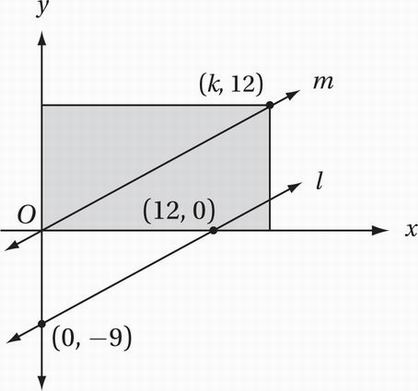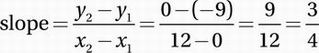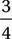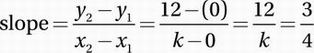# SAT Math Multiple Choice Question 672: Answer and Explanation

### Test Information

Question: 672

12.Note: Figure not drawn to scale.

In the figure above, if m || l, what is the area, in square units, of the shaded rectangle?

• A. 156
• B. 168
• C. 180
• D. 192

Explanation:

D

Special Topics (coordinate geometry) MEDIUM

First, find the slope of l using the points (0, -9) and (12, 0):Since the two lines are parallel, line m must also have a slope of. Now we can solve for k using the slope equation and the two points on line m, (0, 0) and (k, 12):Cross multiply:

4(12) = 3(k)

Simplify:

48 = 3k

Divide by 3:

16 = k

Notice that the coordinates of the point (16, 12) correspond to the width and the length of the rectangle, respectively. Therefore, the area of the rectangle is 16 × 12 = 192 square units.